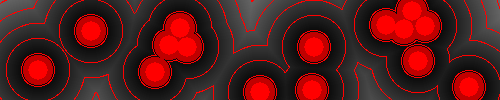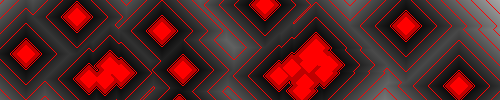BrushName

 Silhouette Isolines Cycle Stroke
Contrast
Brushes
Brush sizeEuclidean
Manhattan
Chessboard
Original

# Meijster distance

A General Algorithm for Computing Distance Transforms in Linear Time
The Meijster distance computes a distance field from a boolean image. A boolean image is made up of zeros and ones, where zero means background (empty space) and one foreground. The resulting distance field is an image whose pixels store distance to foreground.

A distance field can be used for stroking shapes, computing Voronoi diagrams, pathfinding, or performing mathematical morphology operators. The distance field changes depending on the distance function used. Each distance function uses a different shape to compute distance, hold down option to reveal it.Euclidean distance
This is the most commonly used distance function. Given a distance field (x,y) and an image (i,j) the distance field stores the euclidean distance : `sqrt((x-i)2+(y-j)2)`

Pick a point on the distance field, draw a circle using that point as center and the distance field value as radius. The circle will hit the closest foreground point.Manhattan distance (Taxicab geometry)
The distance field stores the Manhattan distance : `abs(x-i)+abs(y-j)`

Pick a point on the distance field, draw a diamond (rhombus) using that point as center and the distance field value as radius. The diamond will hit the closest foreground point.Chessboard distance (Chebyshev)
The distance field stores the chessboard distance : `max(abs(x-i),abs(y-j))`

Pick a point on the distance field, draw a square using that point as center and the double distance field value as edge length. The square will hit the closest foreground point.

## Painting

Left mouse button to paint, shift+left mouse button to clear.

Brushes
Use each brush with its matching transform to recreate the pictures above.

• Circle matches Euclidean
• Diamond matches Manhattan
• Rectangle matches ChessboardUsing the Euclidean distance, paint points with a small brush and up contrast to get Voronoi lookalikes.

## Code

Source is on Github.
PARMANOIR